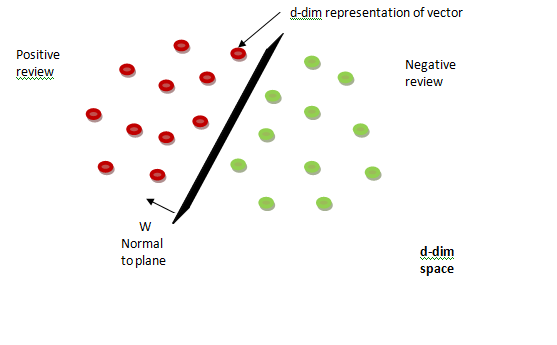# Why convert text to vector in NLP?# Why convert text to vector in NLP?

Text is most unstructured form of data available to us. We can’t predict anything from data when they are in text form. That’s why we have to convert text into vector form so that we can do mathematical operation on vector and predict something from given data.

We are going to convert text(English words and sentences) into numerical vector. Let’s say we have a review text, in the given review text each unique word will represent a unique dimension.

Let’s assume that we have 100 reviews in which 50 are positive and 50 are negative.Each review either positive or negative is going to represent in the form of vector. Now we have to distinguish between positive or negative reviews.

We got review vectors in d-dim space in which each review is a d-dim representation of a vector.Now, we have to find a plane by which we can distinguish between positive and negative reviews.

When we get wTxi>0 then review(ri) is positive.

When we get wTxi<0 then review(ri) is negative.

Let’s take three review r1,r2,r3. Assume r1,r2 are positive reviews or similar reviews and r3 is negative review or dissimilar than r1,r2.

Now the question arises how are we able to find out r1 & r2 are similar and r1 & r3 are dissimilar?

As we are using vector, we can find out the distance between vectors. After finding out the distance if we get distance(v1,v2)<distance(v1,v3) then we can say that similarity(r1,r2)>similarity(r1,r3).

Where, v1 and v2 are vector representation of review r1 and r2. Now we got an idea why we are using vector